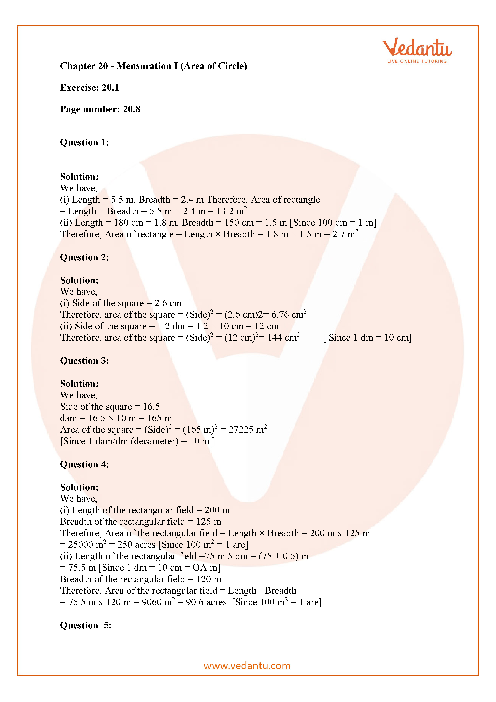RD Sharma Class 7 Maths Solutions Chapter 20 - Mensuration-I

RD Sharma Solutions for Class 7 Maths Chapter 20 - Mensuration I (Area of Circle)

RD Sharma Solutions For Class 7 Maths Chapter 20 PDF  is the best study material for students who find it difficult to solve complex problems. From the links given, students can refer to and download the PDF of RD Sharma Class 7 Solutions Chapter 20. With clear step-by-step explanations, RD Class 7 Maths Chapter 20 Solutions Mensuration is given here. Among Class 7 students, RD Class 7 Maths Chapter 20 Solutions for Mensuration are extremely common for quickly completing their homework and preparing for exams.

Do you need help with your Homework? Are you preparing for Exams?
Study without Internet (Offline)Class 7 RD Sharma Textbook Solutions Chapter 20 - Mensuration I (Area of Circle)

Important Topics of RD Sharma Class 7 Solutions Chapter 20:

Following are some of the essential themes of RD Sharma Class 7 Solutions Chapter 20: Area measurement units, millimetre square, centimetre square, decimeter square, meter square, hectare, kilometre square, perimeter and area of rectangles and squares, area of rectangles, parallelogram, and rhombus areas, and the triangle's area.

We have provided step by step solutions for all exercise questions given in the pdf of Class 7 RD Sharma Chapter 20 - Mensuration I (Area of Circle). All the Exercise questions with solutions in Chapter 20 - Mensuration I (Area of Circle) are given below:

Exercise 20.1

Exercise 20.2

Exercise 20.3

Exercise 20.4

Preparation Tips

• Choose a spot where you can study comfortably, with proper ventilation, lighting, and minimal distractions. Aim to keep your study location clean.

• Mnemonics are methods of memory that help us recall knowledge by associating it with pictures, phrases, or words. There are many ways in which you can construct mnemonics, such as connecting a notion to a visual image.

Conclusion

RD Sharma Solutions For Class 7 Maths Chapter 20 is a relatively simple chapter that, if determined and diligently practised, can be thoroughly learned by the student in a matter of hours. RD Sharma Class 7 Mensuration Solutions will allow learners to study in no time for their exam. This chapter will clarify all the perimeter and area definitions of other plane figures in a detailed way, such as quadrilaterals. In addition, the definition of surface area and volume of solids, such as the cube, cuboid, and cylinder, will be stressed.

1. What is Two-Dimensional and Three-Dimensional Mensuration?

Ans: Mensuration 2D deals specifically with perimeter and region issues. The form is two-dimensional, such as triangles, squares, rectangles, circles, parallelograms, etc. Mensuration 3D addresses forms such as squares, cuboids, spheres, etc. Generally, the problems are based on volume and surface area.

2. Why is the Chapter Mensuration Needed?

Ans: Mensuration can be clarified as a measurement act. We all exist in a three-dimensional universe. In primary as well as secondary school mathematics, the principle of measurement plays an important role. In addition, measurement has a strong association with our daily lives. For both 3D and 2D objects, we learn to do so while learning to measure objects.

3. Who is Known as the Father of Mensuration?

Ans: Leonard Digges is called the father of measurement. Through his writings in English on surveying, cartography, and military engineering, Leonard Digges was a well-known English mathematician and surveyor, credited with the invention of the theodolite, and a great popularizer of science.SHARETWEETSHARESUBSCRIBE